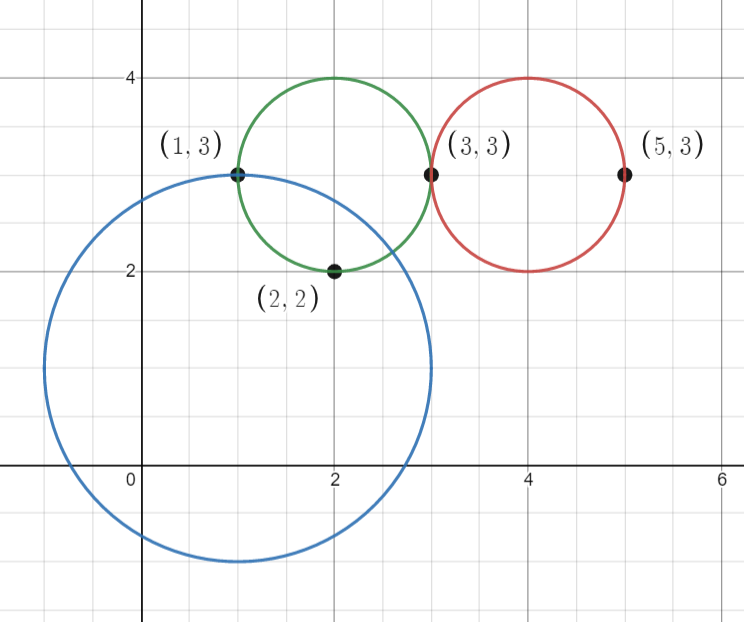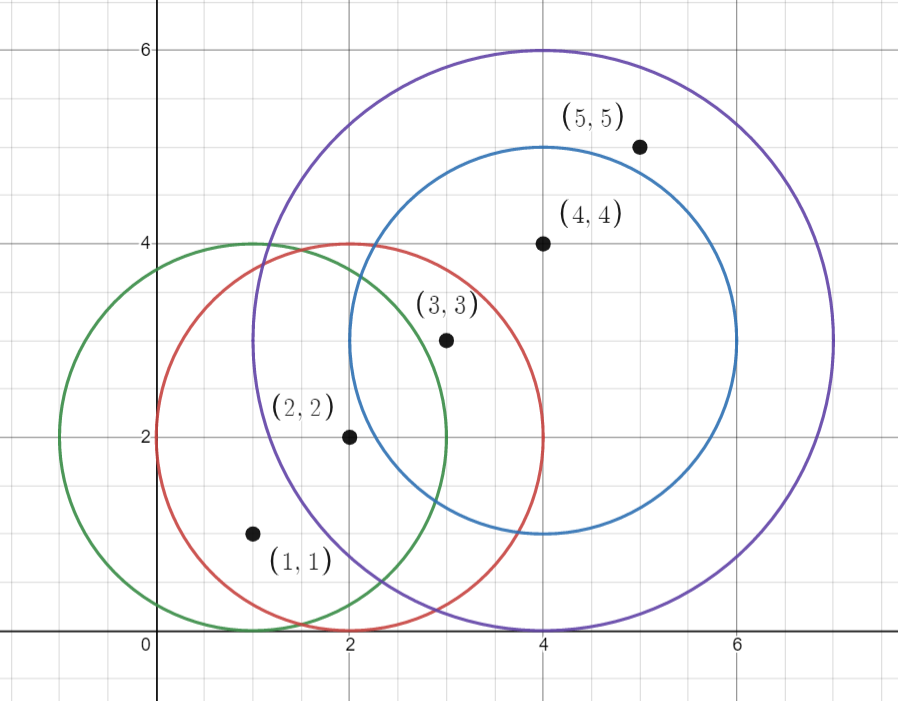Formatted question description: https://leetcode.ca/all/1828.html

# 1828. Queries on Number of Points Inside a Circle

Medium

## Description

You are given an array points where points[i] = [x_i, y_i] is the coordinates of the i-th point on a 2D plane. Multiple points can have the same coordinates.

You are also given an array queries where queries[j] = [x_j, y_j, r_j] describes a circle centered at (x_j, y_j) with a radius of r_j.

For each query queries[j], compute the number of points inside the j-th circle. Points on the border of the circle are considered inside.

Return an array answer, where answer[j] is the answer to the j-th query.

Example 1:Input: points = [[1,3],[3,3],[5,3],[2,2]], queries = [[2,3,1],[4,3,1],[1,1,2]]

Output: [3,2,2]

Explanation: The points and circles are shown above.

queries is the green circle, queries is the red circle, and queries is the blue circle.

Example 2:Input: points = [[1,1],[2,2],[3,3],[4,4],[5,5]], queries = [[1,2,2],[2,2,2],[4,3,2],[4,3,3]]

Output: [2,3,2,4]

Explanation: The points and circles are shown above.

queries is green, queries is red, queries is blue, and queries is purple.

Constraints:

• 1 <= points.length <= 500
• points[i].length == 2
• 0 <= x_i, y_i <= 500
• 1 <= queries.length <= 500
• queries[j].length == 3
• 0 <= x_j, y_j <= 500
• 1 <= r_j <= 500
• All coordinates are integers.

## Solution

For each query, obtain the values x, y and r, and for each point, calculate the squared distance between the query’s center and the point. If the squared distance is less than or equal to r * r, then the point is inside the circle. The number of points inside each circle can be calculated.

class Solution {
public int[] countPoints(int[][] points, int[][] queries) {
int queriesCount = queries.length;
int[] counts = new int[queriesCount];
for (int i = 0; i < queriesCount; i++) {
int[] query = queries[i];
int x = query, y = query, r = query;
int rSquare = r * r;
for (int[] point : points) {
int distanceSquare = getDistanceSquare(point, x, y);
if (distanceSquare <= rSquare)
counts[i]++;
}
}
return counts;
}

public int getDistanceSquare(int[] point, int x, int y) {
return (x - point) * (x - point) + (y - point) * (y - point);
}
}